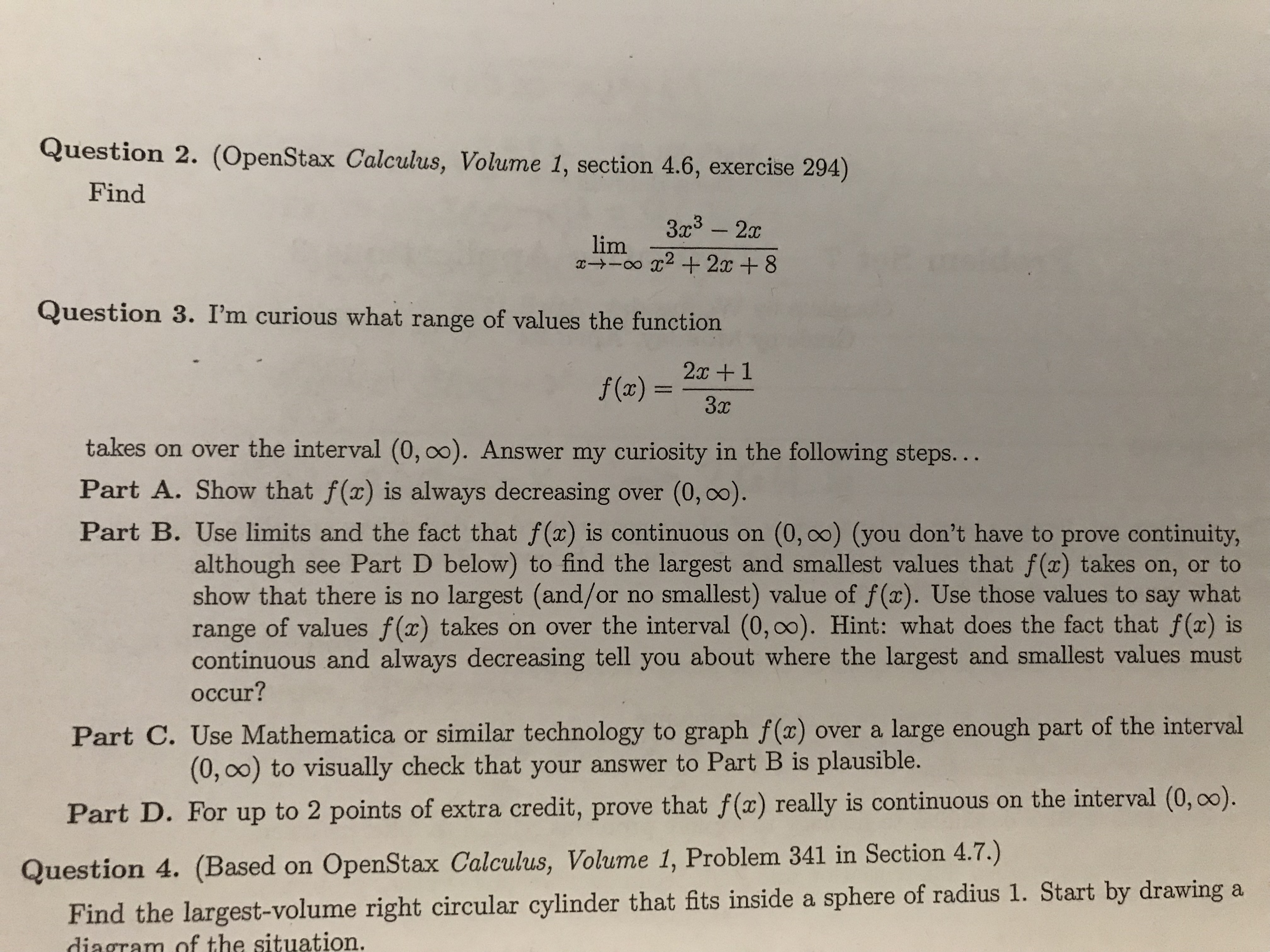# Question 2. (OpenStax Calculus, Volume 1, section 4.6, exercise 294) Find 3a3 -2 lim Question 3. I'm curious what range of values the function 2c+1 takes on over the interval (0, 00). Answer my curiosity in the following steps... Part A. Show that f (x) is always decreasing over (0, oo). Part B. Use limits and the fact that f(x) is continuous on (0, 00) (you don't have to prove continuity, although see Part D below) to find the largest and smallest values that f (x) takes on, or to show that there is no largest (and/or no smallest) value of f(x). Use those values to say what range of values f(x) takes on over the interval (0,00). Hint: what does the fact that f(x) is continuous and always decreasing tell you about where the largest and smallest values must occur? Part C. Use Mathematica or similar technology to graph f(x) over a large enough part of the interval (0, 0o) to visually check that your answer to Part B is plausible. Par t D. For up to 2 points of extra credit, prove that f(a) really is continuous on the interval (0, 00). Question 4. (Based on OpenStax Calculus, Volume 1, Problem 341 in Section 4.7.) Find the largest-volume right circular cylinder that fits inside a sphere of radius 1. Start by drawing a diagram of the situation

Question

How do you do part b to question 3help_outlineImage TranscriptioncloseQuestion 2. (OpenStax Calculus, Volume 1, section 4.6, exercise 294) Find 3a3 -2 lim Question 3. I'm curious what range of values the function 2c+1 takes on over the interval (0, 00). Answer my curiosity in the following steps... Part A. Show that f (x) is always decreasing over (0, oo). Part B. Use limits and the fact that f(x) is continuous on (0, 00) (you don't have to prove continuity, although see Part D below) to find the largest and smallest values that f (x) takes on, or to show that there is no largest (and/or no smallest) value of f(x). Use those values to say what range of values f(x) takes on over the interval (0,00). Hint: what does the fact that f(x) is continuous and always decreasing tell you about where the largest and smallest values must occur? Part C. Use Mathematica or similar technology to graph f(x) over a large enough part of the interval (0, 0o) to visually check that your answer to Part B is plausible. Par t D. For up to 2 points of extra credit, prove that f(a) really is continuous on the interval (0, 00). Question 4. (Based on OpenStax Calculus, Volume 1, Problem 341 in Section 4.7.) Find the largest-volume right circular cylinder that fits inside a sphere of radius 1. Start by drawing a diagram of the situation fullscreen

## Expert Answer

### Want to see this answer and more?

Experts are waiting 24/7 to provide step-by-step solutions in as fast as 30 minutes!*

*Response times may vary by subject and question complexity. Median response time is 34 minutes for paid subscribers and may be longer for promotional offers.
Tagged in
Math
Calculus© 2021 bartleby. All Rights Reserved.